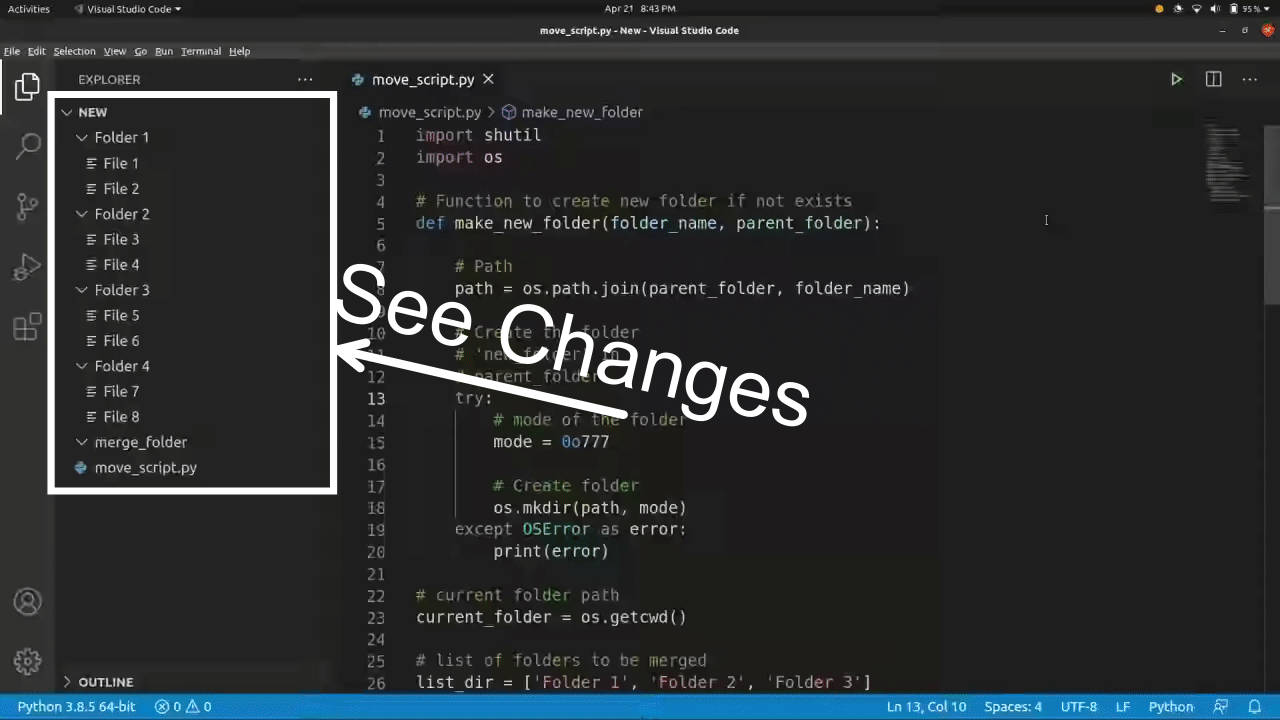Skip to content
Related Articles
How to merge multiple folders into one folder using Python ?
• Last Updated : 28 Apr, 2021

In this article, we will discuss how to move multiple folders into one folder. This can be done using Python’s OS and Shutil module.

### Approach:

1. Get the current directory and the list of the folders you want to merge.
2. Loop through the list of folders and store their content in a list. Here, we have stored them in the dictionary so that we can have the name of the folder as a key and its content as a value list.
3. Specify the folder in which you want to merge all the other folders. If the folder exists then we are good to go but if the folder does not exist then create a new folder.
4. Loop through the dictionary and move all the content of all the listed folders inside the merge folder.

Let’s implement this approach step by step:

Step 1: Below code does the following:

• Get the current directory.
• List all the folders that you want to merge.
• Stores content of all the listed folders in the dictionary with folder name as key and its content as a value list.

## Python3

 `# current folder path``current_folder ``=` `os.getcwd() `` ` `# list of folders to be merged``list_dir ``=` `[``'Folder 1'``, ``'Folder 2'``, ``'Folder 3'``]`` ` `# enumerate on list_dir to get the ``# content of all the folders ans store it in a dictionary``content_list ``=` `{}``for` `index, val ``in` `enumerate``(list_dir):``    ``path ``=` `os.path.join(current_folder, val)``    ``content_list[ list_dir[index] ] ``=` `os.listdir(path)`

Step 2: Creates the merge folder if it does not already exist.

## Python3

 `# Function to create new folder if not exists``def` `make_new_folder(folder_name, parent_folder_path):``     ` `    ``# Path``    ``path ``=` `os.path.join(parent_folder_path, folder_name)``     ` `    ``# Create the folder``    ``# 'new_folder' in``    ``# parent_folder``    ``try``: ``       ` `        ``# mode of the folder``        ``mode ``=` `0o777`` ` `        ``# Create folder``        ``os.mkdir(path, mode) ``         ` `    ``except` `OSError as error: ``        ``print``(error)`` ` `# folder in which all the content ``# will be merged``merge_folder ``=` `"merge_folder"`` ` `# merge_folder path - current_folder ``# + merge_folder``merge_folder_path ``=` `os.path.join(current_folder, merge_folder) `` ` `# create merge_folder if not exists``make_new_folder(merge_folder, current_folder)`

Step 3: Below code does the following:

• Loop through the dictionary with all the folders.
• Now loop through the content of each folder and one by one move them to the merge folder.

## Python3

 `# loop through the list of folders``for` `sub_dir ``in` `content_list:`` ` `    ``# loop through the contents of the``    ``# list of folders``    ``for` `contents ``in` `content_list[sub_dir]:`` ` `        ``# make the path of the content to move ``        ``path_to_content ``=` `sub_dir ``+` `"/"` `+` `contents  `` ` `        ``# make the path with the current folder``        ``dir_to_move ``=` `os.path.join(current_folder, path_to_content )`` ` `        ``# move the file``        ``shutil.move(dir_to_move, merge_folder_path)`

Complete Code:

## Python3

 `import` `shutil``import` `os`` ` ` ` `# Function to create new folder if not exists``def` `make_new_folder(folder_name, parent_folder):``     ` `    ``# Path``    ``path ``=` `os.path.join(parent_folder, folder_name)``     ` `    ``# Create the folder``    ``# 'new_folder' in``    ``# parent_folder``    ``try``: ``        ``# mode of the folder``        ``mode ``=` `0o777`` ` `        ``# Create folder``        ``os.mkdir(path, mode) ``    ``except` `OSError as error: ``        ``print``(error)`` ` `# current folder path``current_folder ``=` `os.getcwd() `` ` `# list of folders to be merged``list_dir ``=` `[``'Folder 1'``, ``'Folder 2'``, ``'Folder 3'``]`` ` `# enumerate on list_dir to get the ``# content of all the folders ans store ``# it in a dictionary``content_list ``=` `{}``for` `index, val ``in` `enumerate``(list_dir):``    ``path ``=` `os.path.join(current_folder, val)``    ``content_list[ list_dir[index] ] ``=` `os.listdir(path)`` ` `# folder in which all the content will``# be merged``merge_folder ``=` `"merge_folder"`` ` `# merge_folder path - current_folder ``# + merge_folder``merge_folder_path ``=` `os.path.join(current_folder, merge_folder) `` ` `# create merge_folder if not exists``make_new_folder(merge_folder, current_folder)`` ` `# loop through the list of folders``for` `sub_dir ``in` `content_list:`` ` `    ``# loop through the contents of the ``    ``# list of folders``    ``for` `contents ``in` `content_list[sub_dir]:`` ` `        ``# make the path of the content to move ``        ``path_to_content ``=` `sub_dir ``+` `"/"` `+` `contents  `` ` `        ``# make the path with the current folder``        ``dir_to_move ``=` `os.path.join(current_folder, path_to_content )`` ` `        ``# move the file``        ``shutil.move(dir_to_move, merge_folder_path)`

Folder structure before running the above program.

```Folder 1
File 1
File 2
Folder 2
File 3
File 4
Folder 3
File 5
File 6
Folder 4
File 7
File 8
merge_folder (Empty)
move_script.py```

Folder structure after running the above program.

```Folder 1 (Empty)
Folder 2 (Empty)
Folder 3 (Empty)
Folder 4 (Untouched)
File 7
File 8
merge_folder
File 1
File 2
File 3
File 4
File 5
File 6
move_script.py```

Program in Working :Attention geek! Strengthen your foundations with the Python Programming Foundation Course and learn the basics.

To begin with, your interview preparations Enhance your Data Structures concepts with the Python DS Course. And to begin with your Machine Learning Journey, join the Machine Learning – Basic Level Course

My Personal Notes arrow_drop_up